• # 循环神经网路的截断长度 TRAINING_STEPS = 3000 # 循环轮数 BATCH_SIZE = 32 # batch大小 TRAINING_EXAMPLES = 10000 # 训练数据的个数 TESTING_EXAMPLES = 1000 # 测试数据的个数 ...
基础介绍可以参考：
https://blog.csdn.net/lilong117194/article/details/82958326
https://blog.csdn.net/lilong117194/article/details/81978203
tensorflow的编程堆栈示意图：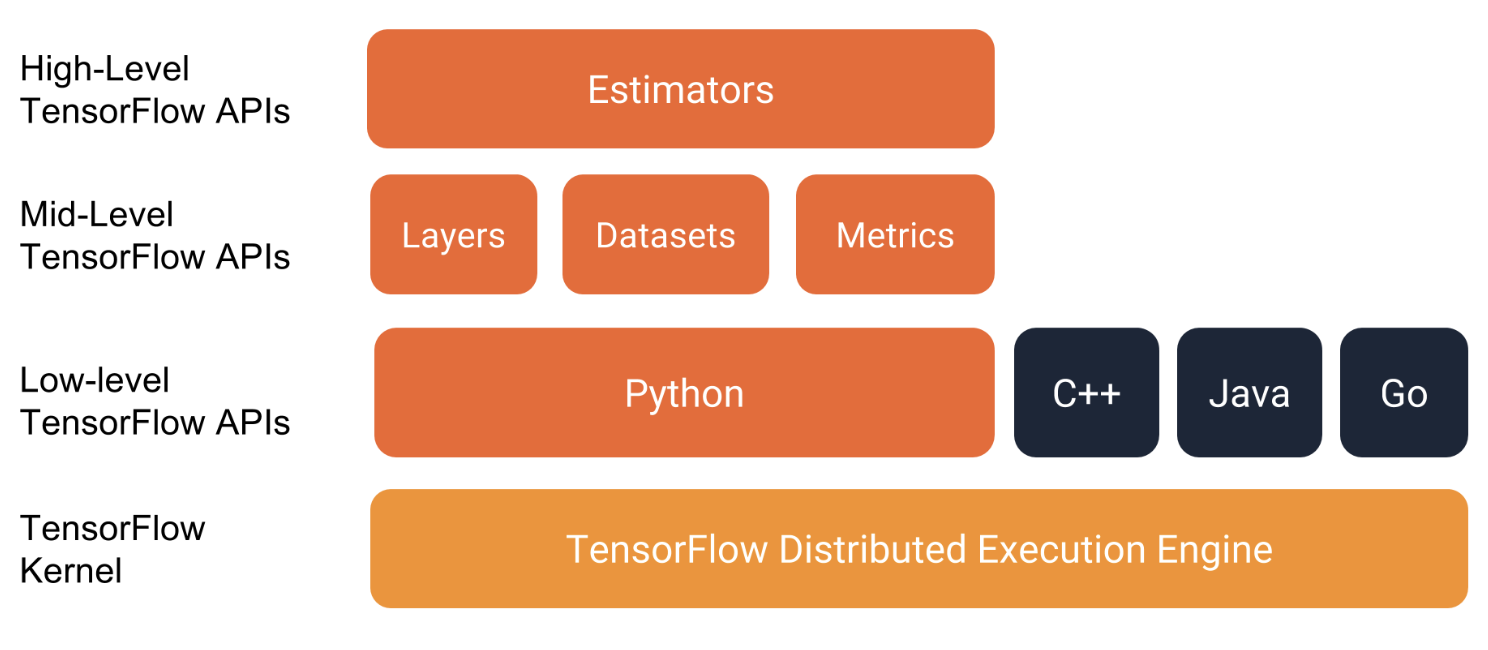强烈建议使用以下API编写TensorFlow程序：

评估器Estimators，代表一个完整的模型。 Estimator API提供方法来训练模型，判断模型的准确性并生成预测。
训练集Datasets，它构建了一个数据输入管道。Datasets API具有加载和操作数据的方法，并将其输入到你的模型中。Datasets API与Estimators API良好地协作。

1. 基于TFlearn的iris分类
下面介绍利用tensorflow的一个高层封装TFlearn。
iris数据集需要通过4个特征来分辨三种类型的植物，iris数据集中总共包含了150个样本，下面是TFlearn解决iris分类问题：
数据集
鸢尾花数据集包含四个特征和一个标签。这四个特征确定了单个鸢尾花的以下植物学特征：

萼片长度
萼片宽度
花瓣长度
花瓣宽度

下表显示了数据集中的三个示例：

萼片长度
萼片宽度
花瓣长度
花瓣宽度
种类（标签）

5.1
3.3
1.7
0.5
0（Setosa）

5.0
2.3
3.3
1.0
1（Versicolor）

6.4
2.8
5.6
2.2
2（virginica）

完整代码：
from sklearn import model_selection
from sklearn import datasets
from sklearn import metrics
import tensorflow as tf
import numpy as np
from tensorflow.contrib.learn.python.learn.estimators.estimator import SKCompat

# 导入TFlearn
learn = tf.contrib.learn

# 自定义softmax回归模型：在给定输入的训练集、训练集标签，返回在这些输入上的预测值、损失值以及训练步骤
def my_model(features, target):
# 将预测目标转化为one-hot编码的形式，共有三个类别，所以向量长度为3
target = tf.one_hot(target, 3, 1, 0)
# 计算预测值及损失函数：封装了一个单层全连接的神经网络
logits = tf.contrib.layers.fully_connected(features, 3, tf.nn.softmax)
loss = tf.losses.softmax_cross_entropy(target, logits)

# 创建模型的优化器，并优化步骤
train_op = tf.contrib.layers.optimize_loss(
loss,                                    # 损失函数
tf.contrib.framework.get_global_step(),  # 获取训练步骤并在训练时更新
learning_rate=0.01)                      # 定义学习率

# 返回给定数据集上的预测结果、损失值以及优化步骤
return tf.arg_max(logits, 1), loss, train_op

# 加载iris数据集，并划分为训练集和测试集
x_train, x_test, y_train, y_test = model_selection.train_test_split(
iris.data, iris.target, test_size=0.2, random_state=0)

#将数据转化成TensorFlow要求的float32格式
x_train, x_test = map(np.float32, [x_train, x_test])

# 封装和训练模型，输出准确率
classifier = SKCompat(learn.Estimator(model_fn=my_model, model_dir="Models/model_1"))
classifier.fit(x_train, y_train, steps=100)

# 预测
y_predicted = [i for i in classifier.predict(x_test)]
# 计算准确度
score = metrics.accuracy_score(y_test, y_predicted)

print('Accuracy: %.2f%%' % (score * 100))


运行结果：
INFO:tensorflow:Using default config.
INFO:tensorflow:Using config: {'_task_type': None, '_task_id': 0, '_cluster_spec': <tensorflow.python.training.server_lib.ClusterSpec object at 0x12a8484e0>, '_master': '', '_num_ps_replicas': 0, '_num_worker_replicas': 0, '_environment': 'local', '_is_chief': True, '_evaluation_master': '', '_train_distribute': None, '_device_fn': None, '_tf_config': gpu_options {
per_process_gpu_memory_fraction: 1.0
}
, '_tf_random_seed': None, '_save_summary_steps': 100, '_save_checkpoints_secs': 600, '_log_step_count_steps': 100, '_session_config': None, '_save_checkpoints_steps': None, '_keep_checkpoint_max': 5, '_keep_checkpoint_every_n_hours': 10000, '_model_dir': 'Models/model_1'}
INFO:tensorflow:Create CheckpointSaverHook.
INFO:tensorflow:Graph was finalized.
INFO:tensorflow:Restoring parameters from Models/model_1/model.ckpt-300
INFO:tensorflow:Running local_init_op.
INFO:tensorflow:Done running local_init_op.
INFO:tensorflow:Saving checkpoints for 300 into Models/model_1/model.ckpt.
INFO:tensorflow:loss = 0.66411054, step = 301
INFO:tensorflow:Saving checkpoints for 400 into Models/model_1/model.ckpt.
INFO:tensorflow:Loss for final step: 0.64143056.
INFO:tensorflow:Graph was finalized.
INFO:tensorflow:Restoring parameters from Models/model_1/model.ckpt-400
INFO:tensorflow:Running local_init_op.
INFO:tensorflow:Done running local_init_op.
Accuracy: 100.00%

下图说明了特征，隐藏层和预测（并未显示隐藏层中的所有节点）：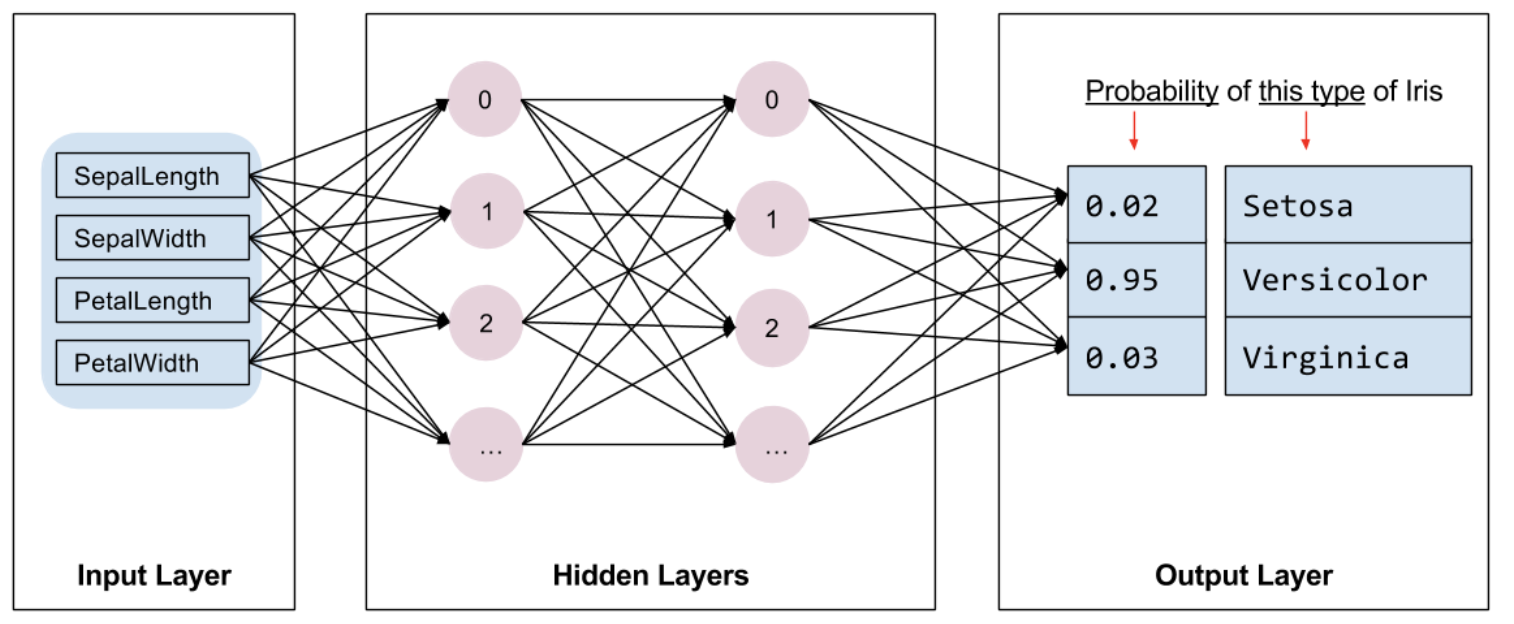下面是评估器(Estimator)编程的基本概念：
Estimator是TensorFlow对完整模型的高级表示。它处理初始化，记录，保存和恢复以及许多其他功能的细节。
Estimator是从tf.estimator.Estimator派生的通用类。 TensorFlow提供了一系列定制的评估器（例如LinearRegressor）来实现常用的ML算法。除此之外，也可以编写自己的定制评估器。但建议在刚开始使用TensorFlow时使用内置的Estimator。在获得内置的Estimator的专业知识后，就可以通过创建定制Estimator来优化模型。
要根据内置的Estimator编写TensorFlow程序，必须执行以下任务：

创建一个或多个输入函数。
定义模型的特征列。
实例化Estimator，指定特征列和各种超参数。
在Estimator对象上调用一个或多个方法，传递适当的输入函数作为数据源。

2. lstm预测正弦函数
下面是利用lstm来实现预测正弦函数sin。
#!/usr/bin/env python3
# -*- coding: utf-8 -*-

import numpy as np
import tensorflow as tf
from tensorflow.contrib.learn.python.learn.estimators.estimator import SKCompat
from tensorflow.python.ops import array_ops as array_ops_

import matplotlib.pyplot as plt

learn = tf.contrib.learn

# 设置神经网络的参数
HIDDEN_SIZE = 30 # LSTM中隐藏的节点个数
NUM_LAYERS = 2  # LSTM的层数

TIMESTEPS = 10  # 循环神经网路的截断长度
TRAINING_STEPS = 3000  # 循环轮数
BATCH_SIZE = 32 # batch大小

TRAINING_EXAMPLES = 10000  # 训练数据的个数
TESTING_EXAMPLES = 1000    # 测试数据的个数
SAMPLE_GAP = 0.01          # 采样间隔

# 定义生成正弦数据的函数
def generate_data(seq):
X = []
y = []
# 序列的第i项和后面的TIMESTEPS-1项合在一起作为输入；第i + TIMESTEPS项作为输
# 出。即用sin函数前面的TIMESTEPS个点的信息，预测第i + TIMESTEPS个点的函数值。
for i in range(len(seq) - TIMESTEPS):
X.append([seq[i: i + TIMESTEPS]])
y.append([seq[i + TIMESTEPS]])
return np.array(X, dtype=np.float32), np.array(y, dtype=np.float32)

# 用正弦函数生成训练和测试数据集合。
test_start = (TRAINING_EXAMPLES + TIMESTEPS) * SAMPLE_GAP   # (10000+10)*0.01=100
test_end = test_start + (TESTING_EXAMPLES + TIMESTEPS) * SAMPLE_GAP # 100+(1000+10)*0.01=110
train_X, train_y = generate_data(np.sin(np.linspace(
0, test_start, TRAINING_EXAMPLES + TIMESTEPS, dtype=np.float32)))
test_X, test_y = generate_data(np.sin(np.linspace(
test_start, test_end, TESTING_EXAMPLES + TIMESTEPS, dtype=np.float32)))

#  定义lstm模型
def lstm_model(X, y, is_training):
# 使用多层的LSTM结构。
cell = tf.nn.rnn_cell.MultiRNNCell([
tf.nn.rnn_cell.BasicLSTMCell(HIDDEN_SIZE)
for _ in range(NUM_LAYERS)])

# 使用TensorFlow接口将多层的LSTM结构连接成RNN网络并计算其前向传播结果。
outputs, _ = tf.nn.dynamic_rnn(cell, X, dtype=tf.float32)
#print(len(outputs))
output = outputs[:, -1, :]

# 对LSTM网络的输出再做加一层全链接层并计算损失。注意这里默认的损失为平均平方差损失函数。
predictions = tf.contrib.layers.fully_connected(
output, 1, activation_fn=None)

# 只在训练时计算损失函数和优化步骤。测试时直接返回预测结果。
if not is_training:
return predictions, None, None

# 计算损失函数。
loss = tf.losses.mean_squared_error(labels=y, predictions=predictions)

# 创建模型优化器并得到优化步骤。
train_op = tf.contrib.layers.optimize_loss(
loss, tf.train.get_global_step(),
return predictions, loss, train_op

"""
定义测试方法
"""
def run_eval(sess, test_X, test_y):
# 将测试数据以数据集的方式提供给计算图。
ds = tf.data.Dataset.from_tensor_slices((test_X, test_y))
ds = ds.batch(1)
X, y = ds.make_one_shot_iterator().get_next()

# 调用模型得到计算结果。这里不需要输入真实的y值。
with tf.variable_scope("model", reuse=True):
prediction, _, _ = lstm_model(X, [0.0], False)

# 将预测结果存入一个数组。
predictions = []
labels = []
for i in range(TESTING_EXAMPLES):
p, l = sess.run([prediction, y])
predictions.append(p)
labels.append(l)

# 计算mse作为评价指标。
predictions = np.array(predictions).squeeze()
labels = np.array(labels).squeeze()
rmse = np.sqrt(((predictions - labels) ** 2).mean(axis=0))
print("Root Mean Square Error is: %f" % rmse)

#对预测的sin函数曲线进行绘图。
plt.figure()
plt.plot(predictions, label='predictions')
plt.plot(labels, label='real_sin')
plt.legend()
plt.show()

"""
执行训练和测试
"""
# 将训练数据以数据集的方式提供给计算图。
print(train_X.shape)
print(train_y.shape)
ds = tf.data.Dataset.from_tensor_slices((train_X, train_y))
ds = ds.repeat().shuffle(1000).batch(BATCH_SIZE)

X, y = ds.make_one_shot_iterator().get_next()

# 定义模型，得到预测结果、损失函数，和训练操作。
with tf.variable_scope("model"):
_, loss, train_op = lstm_model(X, y, True)

with tf.Session() as sess:
sess.run(tf.global_variables_initializer())
# 测试在训练之前的模型效果。
print ("Evaluate model before training.")
run_eval(sess, test_X, test_y)

# 训练模型。
for i in range(TRAINING_STEPS):
_, l = sess.run([train_op, loss])
if i % 1000 == 0:
print("train step: " + str(i) + ", loss: " + str(l))

# 使用训练好的模型对测试数据进行预测。
print ("Evaluate model after training.")
run_eval(sess, test_X, test_y)


运行结果：
(10000, 1, 10)
(10000, 1)
Evaluate model before training.
Root Mean Square Error is: 0.688176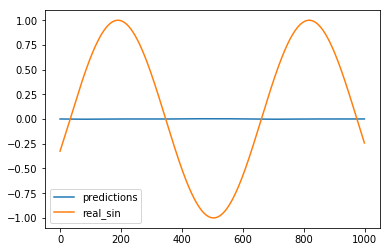train step: 0, loss: 0.5273397
train step: 1000, loss: 0.000585775
train step: 2000, loss: 0.0003093369
Evaluate model after training.
Root Mean Square Error is: 0.007838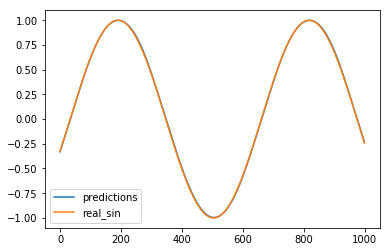预测曲线基本重合与目标曲线。
3. tf.data.Dataset.from_tensor_slices和repeat()、shuffle()、batch()使用

tf.data.Dataset.from_tensor_slices使用

（1）简单数组数列使用
import tensorflow as tf
import numpy as np
## 数据集对象实例化
dataset = tf.data.Dataset.from_tensor_slices(np.array([1.0, 2.0, 3.0, 4.0, 5.0]))
# # 迭代器对象实例化
iterator = dataset.make_one_shot_iterator()
one_element = iterator.get_next()
with tf.Session() as sess:
try:
while True:
print(sess.run(one_element))
except tf.errors.OutOfRangeError:
print("end!")

运行结果：
1.0
2.0
3.0
4.0
5.0
end!

（2）多维数据使用
import tensorflow as tf
import numpy as np

dataset = tf.data.Dataset.from_tensor_slices(np.random.uniform(size=(5, 2)))
print('dataset:',dataset)
iterator = dataset.make_one_shot_iterator()
one_element = iterator.get_next()
with tf.Session() as sess:
try:
while True:
print(sess.run(one_element))
except tf.errors.OutOfRangeError:
print("end!")

运行结果：
dataset: <TensorSliceDataset shapes: (2,), types: tf.float64>
[0.1792582 0.9751422]
[0.19793807 0.33374795]
[0.03978658 0.6808261 ]
[0.98788989 0.07403864]
[0.32259662 0.50171158]
end!

传入的数值是一个矩阵，它的形状为(5, 2)，tf.data.Dataset.from_tensor_slices就会切分它形状上的第一个维度，最后生成的dataset中一个含有5个元素(但这里的元素个数不知道)，每个元素的形状是(2, )，即每个元素是矩阵的一行。
（3）字典使用
import tensorflow as tf
import numpy as np

# 数据集对象实例化
dataset = tf.data.Dataset.from_tensor_slices(
{
"a": np.array([1.0, 2.0, 3.0, 4.0, 5.0]),
"b": np.random.uniform(size=(5, 2))
})

# 迭代器对象实例化
iterator = dataset.make_one_shot_iterator()
one_element = iterator.get_next()

with tf.Session() as sess:
try:
while True:
print(sess.run(one_element))
except tf.errors.OutOfRangeError:
print("end!")


运行结果：
{'a': 1.0, 'b': array([0.04879087, 0.22908515])}
{'a': 2.0, 'b': array([0.11519196, 0.78684246])}
{'a': 3.0, 'b': array([0.86009348, 0.41073689])}
{'a': 4.0, 'b': array([0.80116343, 0.4113549 ])}
{'a': 5.0, 'b': array([0.545203  , 0.23978585])}
end!

对于复杂的情形，比如元素是一个python中的元组或者字典：在图像识别中一个元素可以是｛”image”:image_tensor,”label”:label_tensor｝的形式。 如上所示，这时函数会分别切分”label”中的数值以及”fea”中的数值，最后总dataset中的一个元素就是类似于{ “a”:1.0, “b”:[0.9,0.1] }的形式。
（4）复杂的tuple组合数据
import tensorflow as tf
import numpy as np

dataset = tf.data.Dataset.from_tensor_slices(
(np.array([1.0, 2.0, 3.0, 4.0, 5.0]), np.random.uniform(size=(5, 2)))
)

iterator = dataset.make_one_shot_iterator()
one_element = iterator.get_next()
with tf.Session() as sess:
try:
while True:
print(sess.run(one_element))
except tf.errors.OutOfRangeError:
print("end!")


运行结果：
(1.0, array([0.61708973, 0.73534924]))
(2.0, array([0.64866959, 0.04011806]))
(3.0, array([0.07455173, 0.02324372]))
(4.0, array([0.53580828, 0.19761375]))
(5.0, array([0.63886913, 0.56859266]))
end!


repeat()、shuffle()、batch()使用
Dataset支持一类特殊的操作：Transformation。一个Dataset通过Transformation变成一个新的Dataset。通常我们可以通过Transformation完成数据变换，打乱，组成batch，生成epoch等一系列操作。

（1）batch的使用
import tensorflow as tf
import numpy as np

# 数据集对象实例化
dataset = tf.data.Dataset.from_tensor_slices(
{
"a": np.array([1.0, 2.0, 3.0, 4.0, 5.0]),
"b": np.random.uniform(size=(5, 2))
})

dataset = dataset.batch(2)

# 迭代器对象实例化
iterator = dataset.make_one_shot_iterator()
one_element = iterator.get_next()

with tf.Session() as sess:
try:
while True:
print(sess.run(one_element))
except tf.errors.OutOfRangeError:
print("end!")

运行结果：
{'a': array([1., 2.]), 'b': array([[0.34187133, 0.40294537],
[0.14411398, 0.06565229]])}
{'a': array([3., 4.]), 'b': array([[0.58521367, 0.1550559 ],
[0.39541026, 0.87366261]])}
{'a': array([5.]), 'b': array([[0.10484032, 0.75835755]])}
end!

可以看出每两个数据组成一个batch
（2）shuffle的使用
shuffle的功能为打乱dataset中的元素，它有一个参数buffersize，表示打乱时使用的buffer的大小，建议设置不要太小，一般是1000：
import tensorflow as tf
import numpy as np

dataset = tf.data.Dataset.from_tensor_slices(
{
"a": np.array([1.0, 2.0, 3.0, 4.0, 5.0]),
"b": np.random.uniform(size=(5, 2))
})

dataset = dataset.shuffle(buffer_size=1000)

iterator = dataset.make_one_shot_iterator()
one_element = iterator.get_next()
with tf.Session() as sess:
try:
while True:
print(sess.run(one_element))
except tf.errors.OutOfRangeError:
print("end!")

运行结果：
{'a': 3.0, 'b': array([0.60925085, 0.60532416])}
{'a': 2.0, 'b': array([0.66688525, 0.29313125])}
{'a': 1.0, 'b': array([0.10190033, 0.3020232 ])}
{'a': 4.0, 'b': array([0.20525341, 0.50205214])}
{'a': 5.0, 'b': array([0.4151064 , 0.00696569])}

（3）repeat的使用
repeat的功能就是将整个序列重复多次，主要用来处理机器学习中的epoch，假设原先的数据是一个epoch，使用repeat(2)就可以将之变成2个epoch：
import tensorflow as tf
import numpy as np

dataset = tf.data.Dataset.from_tensor_slices(
{
"a": np.array([1.0, 2.0, 3.0, 4.0, 5.0]),
"b": np.random.uniform(size=(5, 2))
})

dataset = dataset.repeat(3)

iterator = dataset.make_one_shot_iterator()
one_element = iterator.get_next()
with tf.Session() as sess:
try:
while True:
print(sess.run(one_element))
except tf.errors.OutOfRangeError:
print("end!")


运行结果：
{'a': 1.0, 'b': array([0.14897444, 0.444479  ])}
{'a': 2.0, 'b': array([0.10113141, 0.89834262])}
{'a': 3.0, 'b': array([0.18748515, 0.95026317])}
{'a': 4.0, 'b': array([0.56672754, 0.26056881])}
{'a': 5.0, 'b': array([0.5487119 , 0.28498332])}
{'a': 1.0, 'b': array([0.14897444, 0.444479  ])}
{'a': 2.0, 'b': array([0.10113141, 0.89834262])}
{'a': 3.0, 'b': array([0.18748515, 0.95026317])}
{'a': 4.0, 'b': array([0.56672754, 0.26056881])}
{'a': 5.0, 'b': array([0.5487119 , 0.28498332])}
{'a': 1.0, 'b': array([0.14897444, 0.444479  ])}
{'a': 2.0, 'b': array([0.10113141, 0.89834262])}
{'a': 3.0, 'b': array([0.18748515, 0.95026317])}
{'a': 4.0, 'b': array([0.56672754, 0.26056881])}
{'a': 5.0, 'b': array([0.5487119 , 0.28498332])}
end!

参考：
https://zhuanlan.zhihu.com/p/27238630
https://www.cnblogs.com/hellcat/p/8569651.html


展开全文TFlearn iris分类
• ## 循环神经网络

万次阅读 2018-07-23 16:03:08
一、循环神经网络简介 循环神经网络挖掘数据中的时序信息以及语义信息的深度表达能力，在语音识别、语言模型、机器翻译以及时序分析等方面实现了突破。 1、循环神经网络简介 循环神经网络的主要用途是处理和预测...
一、循环神经网络简介

循环神经网络挖掘数据中的时序信息以及语义信息的深度表达能力，在语音识别、语言模型、机器翻译以及时序分析等方面实现了突破。

1、循环神经网络简介
循环神经网络的主要用途是处理和预测序列数据。循环神经网络的来源就是为了刻画一个序列当前的输出与之前信息的关系。从网络结构上，循环神经网络会记忆之前的信息，并利用之前的信息影响后面结点的输出。也就是说，循环神经网络的隐藏层之间的结点是有连接的，隐藏层的输入不仅包括输入层的输出，还包括上一时刻隐藏层的输出。

下图是一个典型的循环神经网络。循环神经网络的主体结构A的输入除了来自输入层xt${x}_{t}$$x_t$，还有一个循环的边来提供上一时刻的隐藏状态ht−1${h}_{t-1}$$h_{t-1}$。在每一时刻，循环神经网络的模块A在读取了xt${x}_{t}$$x_t$和ht−1${h}_{t-1}$$h_{t-1}$之后会生成新的隐藏状态ht${h}_{t}$$h_t$，并产生本时刻的输出ot${o}_{t}$$o_t$。循环神经网络当前的状态ht${h}_{t}$$h_t$是根据上一时刻的状态ht−1${h}_{t-1}$$h_{t-1}$和当前的输入xt${x}_{t}$$x_t$共同决定的。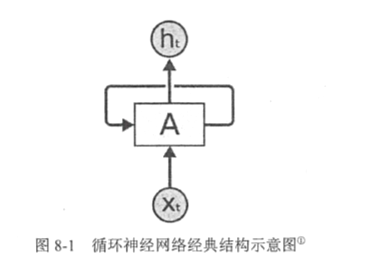在时刻t，状态ht${h}_{t}$$h_t$浓缩了前面序列x0,x1${x}_{0},{x}_{1}$$x_0,x_1$,…,xt−1${x}_{t-1}$$x_{t-1}$的信息，用于作为输出ot${o}_{t}$$o_t$的参考。由于序列长度可以无限长，维度有限的h状态不可能将序列的全部信息都保存下来，因此模型必须学习只保留与后面任务ot,ot+1${o}_{t},{o}_{t+1}$$o_t,o_{t+1}$,…相关的最重要的信息。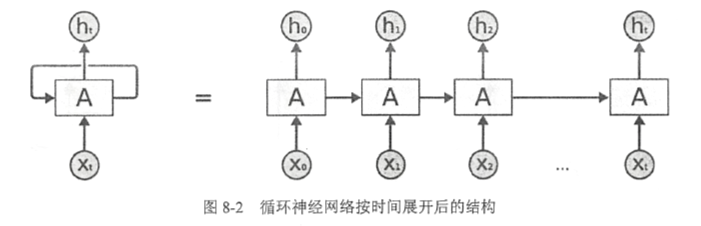上图中，循环神经网络对长度为N的序列展开后，可以视为一个有N个中间层的前馈神经网络。这个前馈神经网络没有循环链接，因此可以直接使用反向传播算法进行训练，而不需要任何特别的优化算法。这样的训练方法称为“沿时间反向传播”，是训练循环神经网络最常见的方法。

对于一个序列数据，可以将这个序列上不同时刻的数据依次传入循环神经网络的输入层，而输出可以是对序列下一时刻的预测，也可以是对当前时刻信息的处理结果（如语音识别结果）。循环神经网络要求每一个时刻都有一个输入，但是不一定每个时刻都需要有输出。

2、循环神经网络前向传播流程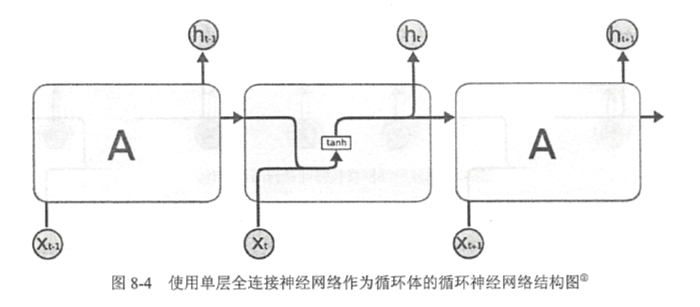上图展示了一个最简单的循环体结构。通过上图介绍循环神经网络前向传播的完整流程。

循环神经网络中的状态是通过一个向量来表示的，这个向量的维度也称为循环神经网络隐藏层的大小，假设其为n。
假设输入向量的维度为x，隐藏层状态的维度为n，那么图中循环体的全连接层神经网络的输入大小为x+n。也就是将上一时刻的状态与当前时刻的输入拼接成一个大的向量作为循环体中神经网络的输入。因为该全连接层的输出为当前时刻的状态，于是输出层的节点个数也为n，循环体中的参数个数为(n+x) x n+n个。

注意：
1、循环体状态与最终输出的维度通常不同，因此为了将当前时刻的状态转化为最终的输出，循环神经网络还需要另外一个全连接神经网络类完成这个过程。这和卷积神经网络中最后的全连接层的意义是一样的。
2、在得到循环神经网络的前向传播结果之后，可以和其他神经网络类似地定义损失函数。循环神经网络唯一的区别在于因为它每个时刻都有一个输出，所以循环神经网络的总损失为所有时刻（或部分时刻）上的损失函数的总和。

以下代码实现了简单的循环神经网络前向传播的过程：

import numpy as np

X=[1,2]
state=[0.0,0.0]
# 分开定义不同输入部分的权重以方便操作
w_cell_state=np.asarray([[0.1,0.2],[0.3,0.4]])
w_cell_input=np.asarray([0.5,0.6])
b_cell=np.asarray([0.1,-0.1])

# 定义用于输出的全连接层参数
w_output=np.asarray([[1.0],[2.0]])
b_output=0.1

# 按照时间顺序执行循环神经网络的前向传播过程
for i in range(len(X)):
# 计算循环体中的全连接层神经网络
before_activation=np.dot(state,w_cell_state)+X[i]*w_cell_input+b_cell
state=np.tanh(before_activation)
# 根据当前时刻状态计算最终输出
final_output=np.dot(state,w_output)+b_output
# 输出每个时刻的信息
print("before activation:%f" % before_activation)
print("state:%f" % state)
print("output:%f" % final_output)

运行以上程序可以看到输出：

before activation:[0.6 0.5]
state:[0.53704957 0.46211716]
output:[1.56128388]
before activation:[1.2923401 1.39225678]
state:[0.85973818 0.88366641]
output:[2.72707101]

和其他神经网络类似，在定义完损失函数之后，使用类似地优化框架，Tensorflow就可以自动完成模型训练的过程。需要指出的是：理论上循环神经网络可以支持任意长度的序列，然而在实际训练过程中，如果序列过长，一方面会导致训练时出现梯度消失和梯度爆炸的问题；另一方面，展开后的循环神经网络会占用过大的内存，所以实际中会规定一个最大长度，当序列长度超过规定长度后会对序列进行截断。

二、长短时记忆网络（LSTM）结构

当前预测位置和相关信息之间的文本间隔不断增大时，简单循环神经网络有可能会丧失学习到距离如此远的信息的能力，或者在复杂语言场景中，有用信息的间隔有大有小、长短不一，循环神经网络的性能也会受到限制。

长短时记忆模型（long short-term memory，LSTM）的设计就是为了解决这个问题。采用LSTM结构的循环神经网络比标准的循环神经网络表现更好。与单一tanh循环体结构不同，LSTM是一种拥有三个“门”结构的特殊网络结构。
1、LSTM结构
LSTM靠一些“门”的结构让信息有选择性地影响循环神经网络中每个时刻的状态。所谓“门”结构就是一个使用sigmoid神经网络和一个按位做乘法的操作，这两个操作合在一起就是一个“门”的结构。使用sigmoid作为激活函数的全连接神经网络层会输出一个0到1之间的数值，描述当前输入有多少信息量可以通过这个结构。当门打开时（sigmoid神经网络层输出为1时），全部信息都可以通过；当门关上时（sigmoid神经网络层输出为0时），任何信息都无法通过。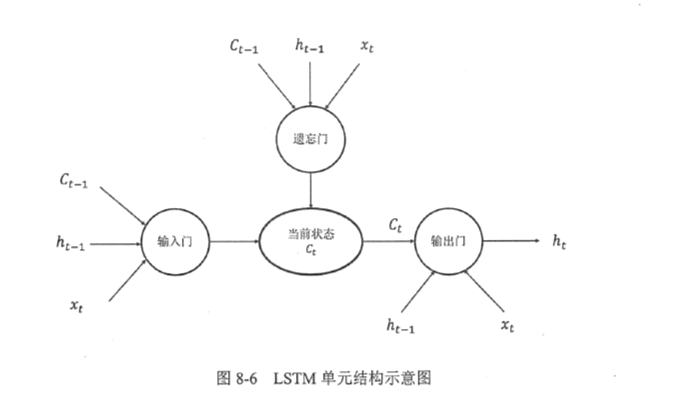上图中“遗忘门”和“输入门”可以使神经网更有效的保存长期记忆。“遗忘门”的作用是让循环神经网络忘记之前没有用的信息。“遗忘门”会根据当前输入xt${x}_{t}$$x_t$和上一时刻输出ht−1${h}_{t-1}$$h_{t-1}$决定哪一部分记忆需要被遗忘。

（1）假设状态c的维度为n。“遗忘门”会根据当前输入xt${x}_{t}$$x_t$和上一时刻输出ht−1${h}_{t-1}$$h_{t-1}$计算一个维度为n的向量f=sigmoid(W1x+W2h)$f=sigmoid\left({W}_{1}x+{W}_{2}h\right)$$f=sigmoid(W_1x+W_2h)$，它的每一维度上的值都在（0,1）范围内，再将上一时刻的状态ct−1${c}_{t-1}$$c_{t-1}$与f向量按位相乘，那么f取值接近0的维度上的信息就会被“忘记”，而f取值接近1的维度上的信息会被保留。
比如文章中先介绍了某地原来是绿水蓝天，后来被污染了。于是在看到被污染之后，循环神经网络应该“忘记”之前绿水蓝天的状态。
（2）在循环网络“忘记”了部分之前的状态后，它还需要从当前的输入补充最新的记忆。这个过程由“输入门”完成。“输入门”会根据xt${x}_{t}$$x_t$和ht−1${h}_{t-1}$$h_{t-1}$决定哪些信息加入到状态ct−1${c}_{t-1}$$c_{t-1}$中生成新的状态ct${c}_{t}$$c_t$。
比如当看到文章中提到环境被污染之后，模型需要将这个信息写入新的状态。
**（3）**LSTM结构在计算得到新的状态ct${c}_{t}$$c_t$后需要产生当前时刻的输出，这个过程由“输出门”完成。“输出门”会根据最新的状态ct${c}_{t}$$c_t$、上一时刻的输出ht−1${h}_{t-1}$$h_{t-1}$和当前的输入xt${x}_{t}$$x_t$来决定该时刻的输出ht${h}_{t}$$h_t$。
比如当前的状态为被污染，那么“天空的颜色”后面的单词很有可能是“灰色的”。

2、LSTM前向传播过程
具体LSTM每个“门”的公式定义如下：

z=tanh(Wz[ht−1,xt])$z=tanh\left({W}_{z}\left[{h}_{t-1},{x}_{t}\right]\right)$$z=tanh(W_z[h_{t-1},x_t])$         输入值
i=sigmoid(Wi[ht−1,xt])$i=sigmoid\left({W}_{i}\left[{h}_{t-1},{x}_{t}\right]\right)$$i=sigmoid(W_i[h_{t-1},x_t])$      输入门
f=sigmoid(Wf[ht−1,xt])$f=sigmoid\left({W}_{f}\left[{h}_{t-1},{x}_{t}\right]\right)$$f=sigmoid(W_f[h_{t-1},x_t])$      遗忘门
o=sigmoid(Wo[ht−1,xt])$o=sigmoid\left({W}_{o}\left[{h}_{t-1},{x}_{t}\right]\right)$$o=sigmoid(W_o[h_{t-1},x_t])$      输出门
ct=f⋅ct−1+i⋅z${c}_{t}=f\cdot {c}_{t-1}+i\cdot z$$c_t=f\cdot c_{t-1}+i\cdot z$                         新状态
ht=o⋅tanhct${h}_{t}=o\cdot tanh{c}_{t}$$h_t=o \cdot tanhc_t$                           输出
其中Wz${W}_{z}$$W_z$$W_i$Wf$\mathrm{}{W}_{f}$$\W_f$$W_o$是4个维度为[2n,n]的参数矩阵。

3、Tensorflow中LSTM结构的实现
以下代码展示了在Tensorflow中实现使用LSTM结构的循环神经网络的前向传播过程：

# 定义一个LSTM结构。在Tensorflow中通过一句简单的命令就可以实现一个完整的LSTM结构
# LSTM中使用的变量也会在该函数中自动被声明
lstm=tf.nn.rnn.cell.BasicLSTMCell(lstm_hidden_size)

# 将LSTM中的状态初始化为全0数组。BasicLSTMCell类提供了zero_state函数来生成全0初始状态。state是一个
# 包含两个张量的LSTMStateTuple类，其中state.c和state.h分别对应了上述c状态和h状态
# 和其他神经网络类似，在优化循环神经网络时，每次也会使用一个batch的训练样本
# 在以下代码中，batch_size给出了一个batch的大小
state=lstm.zero_state(batch_size,tf.float32)

# 定义损失函数
loss=0.0

# 虽然在测试时循环神经网络可以处理任意长度的序列，但是在训练中为了将循环神经网络展开成前馈神经网络，我们需要
# 知道训练数据的序列长度。在以下代码中，用num_steps来表示这个长度。后面会介绍使用dynamic_rnn动态处理变长
# 序列的方法
for i in range(num_steps):
# 在第一时刻声明LSTM结构中使用的变量，在之后的时刻都需要复用之前定义好的变量。
if i>0:
tf.get_variable_scpoe.reuse_variables()
# 每一步处理时间序列中的一个时刻。将当前输入current_input和前一时刻状态state(h_t-1和c_t-1)传入定义
# 的LSTM结构可以得到当前LSTM的输出lstm_output(ht)和更新后状态state(ht和ct)。lstm_output用于输出
# 给其他层，state用于输出给下一时刻，他们在dropout等方面可以有不同的处理方式。
lstm_output,state=lstm(current_input,state)
# 将当前时刻LSTM结构的输出传入一个全连接层得到最后的输出
final_output=fully_connected(lstm_output)
# 计算当前时刻输出的损失
loss+=calc_loss(final_output,expected_output)
# 使用常规神经网络的方法训练模型

！！！！
注意：
在第一时刻声明LSTM结构中使用的变量，在之后的时刻都需要复用之前定义好的变量
！！！！

三、循环神经网络的变种

1、双向循环神经网络

在经典的循环神经网络中，状态和传输是从前往后单向的。然而在有些问题中，当前时刻的输出不仅和之前的状态有关，也和之后的状态相关。这时需要使用双向循环神经网络（bidirectional RNN）来解决这个问题。双向循环神经网络是由两个独立的循环神经网络叠加在一起组成的。输出由这两个循环神经网络的输出拼接而成。下图展示了一个双向循环神经网络的结构：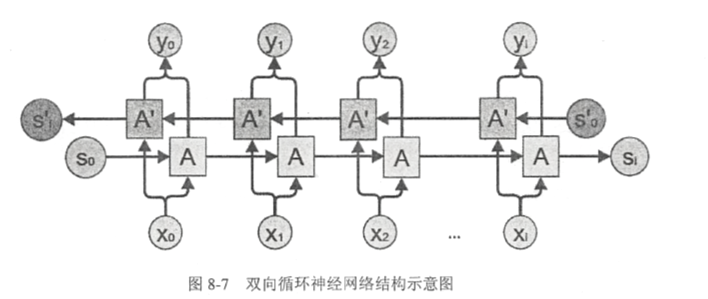在每一个时刻t，输入会同时提供给这两个方向相反的循环神经网络、两个独立的网络独立进行计算，各自产生该时刻的新状态和输出，而双向循环神经网络的最终输出是这两个单向循环神经网络的输出的简单拼接。两个循环神经网络除方向不同以外，其余结构完全对称。每一个网络中的循环体可以自由选用任意结构，RNN或LSTM。

2、深层循环神经网络

深层循环神经网络（Deep RNN）是循环神经网络的另一种变种。为了增强模型的表达能力，可以在网络中设置多个循环层，将每层循环网络的输出传给下一层进行处理。下图展示了一个深层循环神经网络的结构：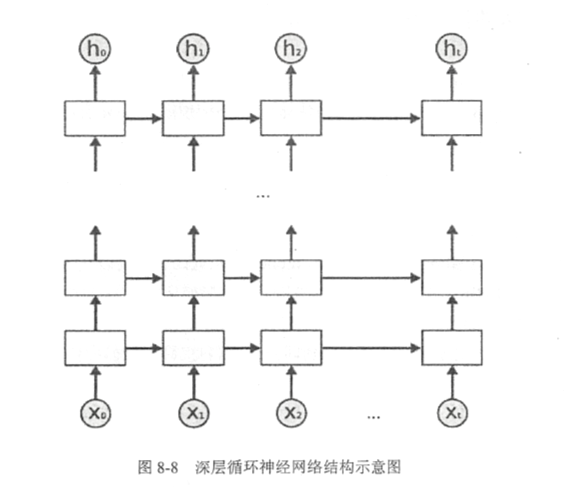在一个L层的深层循环网络中，每一时刻的输入xt${x}_{t}$$x_t$到输出ot${o}_{t}$$o_t$之间有L个循环体，网络因此可以从输入中抽取更加高层的信息。和卷积神经网络类似，每一层的循环体中参数是一致的，而不同层中的参数可以不同。为了更好支持深层循环神经网络，Tensorflow中提供了MultiRNNCell类来实现深层循环神经网络的前向传播过程。

# 定义一个基本的LSTM结构作为循环体的基础结构。深层循环神经网络也支持使用其他的循环体结构
lstm_cell=tf.nn.rnn.cell.BasicLSTMCell
# 通过MUltiRNNCell类实现深层循环神经网络中每一个时刻的前向传播过程。其中number_of_layers表示有多少层，
# 即从x_t到o_t需要经过多少个LSTM结构。
stacked_lstm=tf.nn.rnn_cell.MultiRNNCell([lstm_cell(lstm_size) for _ in range(number_of_layers)])

# 和经典的循环神经网络一样，可以通过zero_state函数来获取初始状态
state=stacked_lstm.zero_state(batch_size,tf.float32)

# 计算每一时刻的前向传播结果
for i in range(len(num_steps)):
if i>0:
tf.get_variable_scope().reuse_variables()
stacked_lstm_output,state=stacked_lstm(current_input,state)
final_output=fully_connected(stacked_lstm_output)
loss+=calc_loss(final_output,expected_output)

Tensorflow只需要在BasicLSTMCell的基础上再封装一层MultiRNNCell就可以非常容易地实现深层循环神经网络了。

3、循环神经网络dropout

在循环神经网络中使用dropout可以让神经网络更加健壮。类似在卷机神经网络只在最后的全连接层中使用dropout，循环神经网络一般只在不同层循环体结构之间使用dropout，而不在同一层的循环体结构之间使用。即从时刻t-1传递到时刻t时，循环神经网络不会进行状态的dropout；而在同一时刻t中，不同层循环体之间会使用dropout。

下图展示了循环神经网络使用dropout的方法。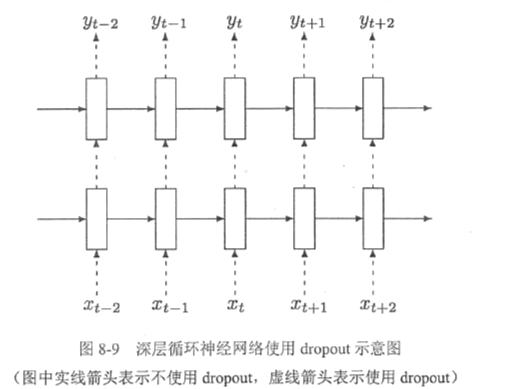假设要从t-2时刻的输入xt−2${x}_{t-2}$$x_{t-2}$传递到t+1时刻的输出yt+1${y}_{t+1}$$y_{t+1}$，那么xt−2${x}_{t-2}$$x_{t-2}$将首先传入第一层循环体结构，这个过程会使用dropout，但是从t-2时刻的第一层循环体结构传递到第一层的t-1、t、t+1时刻不会使用dropout。在t+1时刻的第一层循环体结构传递到同一时刻内更高层的循环体结构时，会再次使用dropout。

在Tensorflow中，使用tf.nn.rnn_cell.DropoutWrapper类可以很容易实现dropout功能。以下代码展示了如何在Tensorflow中实现带dropout的循环神经网络。

# 定义LSTM结构
lstm_cell=tf.nn.rnn_cell.BasicLSTMCell

# 使用DropoutWrapper类来实现dropout功能。该类通过两个参数来控制dropout的概率，一个参数为
# input_keep_prob，它可以用来控制输入的dropout概率；另一个参数为output_Keep_prob，它可以用来
# 控制输出的dropout概率
# 在使用了DropoutWrapper的基础上定义MultiRNNCell
stacked_lstm=rnn_cell.MultiRNNCell([tf.nn.rnn_Cell.DropoutWrapper(lstm_cell(lstm_size)) for _ in range(number_of_layers)])

四、循环神经网络样例应用

以时间预测为例，利用循环神经网络实现对函数sin x取值的预测

# -*- coding: utf-8 -*-

import numpy as n
import yensorflow as tf

# 加载matplotlib工具包，使用该工具包可以对预测的sin函数曲线进行绘图
import matplotlib as mpl
mpl.use('Agg')
from matplotlib import pyplot as plt

HIDEN_SIZE=30  # LSTM中隐藏节点的个数
NUM_LAYERS=2   # LSTM的层数

TIMESTEPS=10   # 循环神经网络的训练序列长度
TRAINING_STEPS=10000 # 训练轮数
BATCH_SIZE=32  # batch的大小

TRAINING_EXAMPLES=10000  # 训练数据大小
TESTING_EXAMPLES=1000    # 测试数据个数
SAMPLE_GAP=0.01          # 采样间隔

def generate_data(seq):
X=[]
y=[]
# 序列的第i项和后面的TIMESTEPS-1项合在一起作为输入；第i+TIMESTEPS项作为输出。即用sin函数前面的
# TIMESTEPS个点的信息，预测第i+TIMESTEPS个点的函数值
for i in range(len(seq)-TIMESTEPS)
X.append([seq[i:i+TIMESTEPS]])
y.append([seq[i+TIMESTEPS]])
return np.array(X,dtype=np.float32),np.array(y,dtype=np.float32)

def lstm_model(X,y,is_training)
# 使用多层的LSTM结构
cell=tf.nn.rnn_cell.MultiRNNCell([tf.nn.rnn_cell.BasicLSTMCell(HIDDEN_SIZE) for _ in range(NUM_LAYERS)])
# 使用Tensorflow接口将多层LSTM结构连接成RNN网络并计算其前向传播结果
outputs,_=tf.nn.dynamic_rnn(cell,X,dtype=tf.float32)
# outputs是顶层LSTM在每一步的输出结果，维度是[batch_size,time,HIDDEN_SIZE]在本问题中只关注最后一
# 个时刻的输出结果
output=outputs[:,-1,:]
# 对LSTM网络的输出再做加一层全连接层并计算损失。这里默认的损失为平均平方差损失函数
predictions=tf.contrib.layers.fully_connected(output,1,activation_fn=None)
# 只在训练时计算损失函数和优化步骤。测试时直接返回预测结果
if not is_training:
return predictions,None,None
loss=tf.losses.mean_squared_error(labels=y,predictions=predictions)
# 创建模型优化器并得到优化步骤
return predictions,loss,train_op

def train(sess,train_X,train_y):
# 将训练数据以数据集的方式提供给计算图
ds=tf.data.Dataset.from_tensor_slices((train_X,train_y))
ds=ds.repeat().shuffle(1000).batch(BATCH_SIZE)
X,y=ds.make_one_shot_iterator().get_next()
# 调用模型，得到预测结果、损失函数和训练操作
with tf.variable_scpoe("model"):
predictions,loss,train_op=lstm_model(X,y,True)
# 初始化变量
sess.run(tf.global_variables_initializer())
for i in range(TRAINING_STEPS):
_,l=sess.run([train_op,loss])
if i%100 == 0:
print("train step:"+str(i)+",loss:"+str(l))
def run_eval(sess,test_X,test_y):
# 将测试数据以数据集的方式提供给计算图
ds=tf.data.Dataset.from_tensor_slices((test_X,test_y))
ds=d.batch(1)
X,y=ds.make_one_hot_interator().get_next()

# 调用模型得到计算结果，这里不需要输入真实的y值
with tf.variables_scope("model",reuse=True):
prediction,_,_=lstm_model(X,[0.0],False)
# 将预测结果存入一个数组
predictions=[]
labels=[]
for i in range(TESTING_EXAMPLES):
p,l=sess.run([prediction,y])
predections.append(p)
labels.append(l)
# 计算rmse作为评价指标
predictions=np.array(predictions).squeeze()
labels=np.array(labels).squeeze()
rmse=np.sqrt(((predictions-labels)**2).mean(axis=0))
print("Mean Square Error is:%f"  % rmse)

# 对预测的sin函数曲线进行绘图
plt.figure()
plt.plot(predictions,label='predictions')
plt.plot(labels,label='real_sin')
plt.legend()
plt.show()

# 用正弦函数生成训练和测试数据集合
# numpy.linspace函数可以创建一个等差序列的数组，它常用的参数有3个，第一个参数表示起始值，第二个参数表示
# 终止值，第三个参数表示数列的长度。如linespace(1,10,10)产生的数组是array([1,2,3,4,5,6,7,8,9,10])
test_start=(TRAING_EXAMPLES+TIMESTEPS)*SAMPLE_GAP
test_end=test_start+(TESTING_EXAMPLES+TIMESTEPS)*SAMPLE_GAP
train_X,train_y=generate_data(np.sin(np.linspace(0,test_start,TRAINING_EXAMPLES+TIMESTEPS,dtype=np.float32)))
test_X,test_y=generate_data(np.sin(np.linspace(test_start,test_end,TESTING_EXAMPLES+TIMESTRPS,dtype=np.float32)))

with tf.Session() as sess:
# 训练模型
train(sess,train_X,train_y)
# 使用训练好的模型对测试数据进行预测
run_eval(sess,test_X,test_y)
展开全文LSTM
• 微信公众号  本文同步更新在我的微信公众号里，地址：... ... 目录 1. 基本循环神经网络 2. 双向循环神经网络 2.1 双向循环神经网络的介绍 2.2 双向循环神经网络的训练 ...


本文同步更新在我的微信公众号里，地址：https://mp.weixin.qq.com/s/IPyI2Ee6Kzyv3wFAUN7NOQ

本文同步更新在我的知乎专栏里，地址：https://zhuanlan.zhihu.com/p/43190710

目录

1. 基本循环神经网络

2. 双向循环神经网络

2.1 双向循环神经网络的介绍

2.2 双向循环神经网络的训练

3. 深度循环神经网络

4. 循环神经网络的时间步长和参数共享

4.1 循环神经网络的time steps

4.2 循环神经网络的参数共享

5. 循环神经网络的训练算法（BPTT）

6. RNN的梯度消失和梯度爆炸

6.1 梯度消失

6.2 梯度爆炸

7. RNN的Long-Term依赖问题

8. RNN的几种架构

8.1 1 to 1

8.2 1 to N

8.3 N to 1

8.4 N to N

8.5 N to M

Reference

1. 基本循环神经网络

传统的神经网络模型是从输入层到隐含层再到输出层的全连接，且同层的节点之间是无连接，网络的传播也是顺序的，但这种普通的网络结构对于许多问题却显得无能为力。例如，在自然语言处理中，如果要预测下一个单词，就需要知道前面的部分单词，因为一个句子中的单词之间是相互联系的，即有语义。这就需要一种新的神经网络，即循环神经网络RNN，循环神经网络对于序列化的数据有很强的模型拟合能力。具体的结构为：循环神经网络在隐含层会对之前的信息进行存储记忆，然后输入到当前计算的隐含层单元中，也就是隐含层的内部节点不再是相互独立的，而是互相有消息传递。隐含层的输入不仅可以由两部分组成，输入层的输出和隐含层上一时刻的输出，即隐含层内的节点自连；隐含层的输入还可以由三部分组成，输入层的输出、隐含层上一时刻的输出、上一隐含层的状态，即隐含层内的节点不仅自连还互连。结构如图1所示。

在图1中，可以看到隐含层节点间有消息的相互传递。为了更简单的理解，现在我们将RNN在时间坐标轴上展开成一个全神经网络，如图2所示。例如，对一个包含3个单词的语句，那么展开的网络便是一个有3层的神经网络，每一层代表一个单词。

对于图2的网络，计算过程如下：

表示第步(step)的输入。比如为第二个词的词向量(为第一个词)；

为隐藏层的第t步的状态，它是网络的记忆单元。根据当前输入层的输出与上一时刻隐藏层的状态进行计算，如公式1所示。其中，U是输入层的连接矩阵，W是上一时刻隐含层到下一时刻隐含层的权重矩阵，f(x)一般是非线性的激活函数，如tanh或ReLU。

(1)

是第t步的输出。输出层是全连接层，即它的每个节点和隐含层的每个节点都互相连接，V是输出层的连接矩阵，g(x)是激活函数。
(2)

如果将(1)式循环带入(2)式可得：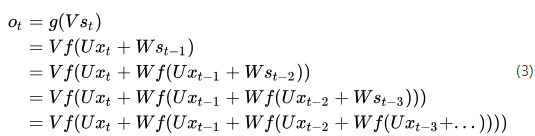由式(3)可以看出，循环神经网络的输出值与前面多个时刻的历史输入值有关，这就是为何循环神经网络能够往前看任意多个输入值的原因，也就是为何循环神经网络能够对序列数据建模的原因。

在图2中，我们展示了一个单向循环神经网络，但是单向循环神经网络也有不足之处。从单向的结构中可以知道它的下一时刻预测输出是根据前面多个时刻的输入共同影响的，而有些时候预测可能需要由前面若干输入和后面若干输出共同决定，这样才会更加准确。

2. 双向循环神经网络

2.1 双向循环神经网络的介绍

对于语言模型来说，很多时候单向循环神经网络表现是不好的，比如下面这句话：

我的手机坏了，我打算____一部新手机。

可以想象，如果我们只看横线前面的词，手机坏了，那么我是打算修一修？换一部新的？还是大哭一场？这些都是无法确定的。但如果我们也看到了横线后面的词是『一部新手机』，那么，横线上的词填『买』的概率就大得多了。

对于上面的语言模型，单向循环神经网络是无法对此进行建模的。因此，我们需要用双向循环神经网络，如图3所示。

从图3中可以看出，双向循环神经网络的隐藏层要保存两个值，一个A参与正向计算，另一个值参与反向计算。我们以的计算为例，推出循环神经网络的一般规律。最终的输出值取决于和  。其计算方式为公式4：

(4)

和 则分别计算为：

(5)

(6)

现在，我们已经可以看出一般的规律：正向计算时，隐藏层的值与 有关；反向计算时，隐藏层的值与 有关；最终的输出取决于正向和反向计算的加和。现在，我们仿照式5和式6，写出双向循环神经网络的计算方法：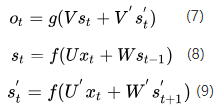从上面三个公式我们可以看到，正向计算和反向计算不共享权重，也就是说U和U'、W和W'、V和V'都是不同的权重矩阵。

2.2 双向循环神经网络的训练

前向传播：

沿着时刻0到时刻i正向计算一遍，得到并保存每个时刻向前隐含层的输出。

沿着时刻i到时刻0反向计算一遍，得到并保存每个时刻向后隐含层的输出。

正向和反向都计算完所有输入时刻后，每个时刻根据向前向后隐含层得到最终输出。

反向传播：

计算所有时刻输出层的损失函数项。

根据所有输出层的损失函数项，使用 BPTT 算法更新向前层。

根据所有输出层的损失函数项，使用 BPTT 算法更新向后层。

3. 深度循环神经网络

上面介绍的基本循环神经网络和双向循环神经网络只有单个隐藏层，有时不能很好的学习数据内部关系，可以通过叠加多个隐藏层，形成深度循环神经网络结构。

Deep （Bidirectional）RNN和Bidirectional RNN相似，只是在每个时刻会有多个隐藏层。在实际中会有更强的学习能力，但也需要更多的训练数据。

4. 循环神经网络的时间步长和参数共享

4.1 循环神经网络的time steps

time steps 就是循环神经网络认为每个输入数据与前多少个陆续输入的数据有联系。例如具有这样一段序列数据 “…ABCDBCEDF…”，当 time steps 为 3 时，在模型预测中如果输入数据为“D”，那么之前接收的数据如果为“B”和“C”则此时的预测输出为 B 的概率更大，之前接收的数据如果为“C”和“E”，则此时的预测输出为 F 的概率更大。

4.2 循环神经网络的参数共享

循环神经网络参数共享指的是：在每一个时间步上，所对应的参数是共享的。参数共享的目的有两个：一、用这些参数来捕获序列上的特征；二、共享参数减少模型的复杂度。

如图2所示，在RNN中每输入一步，每一层各自都共享参数U、V、W。其反映着RNN中每一时刻都在做相同的事情，只是输入不同，因此大大减少了网络中需要学习的参数。

对于RNN的参数共享，我们可以理解为对于一个句子或者文本，参数U可以看成是语法结构、参数W是一般规律，而下一个单词的预测必须是上一个单词和一般规律W与语法结构U共同作用的结果。我们知道，语法结构和一般规律在语言当中是共享的。所以，参数自然就是共享的！

CNN和RNN参数共享的区别：

我们需要记住的是，深度学习是怎么减少参数的，很大原因就是参数共享，而CNN是在空间上共享参数，RNN是在时间序列上共享参数。

循环神经网络中关于参数共享比较好的文章推荐：

【1】YJango的循环神经网络——介绍 - YJango的文章 - 知乎 https://zhuanlan.zhihu.com/p/24720659

【2】全面理解RNN及其不同架构 - Evan的文章 - 知乎 https://zhuanlan.zhihu.com/p/34152808

5. 循环神经网络的训练算法（BPTT）

RNN的反向传播算法为Backpropagation Through Time (BPTT)。让我们先回忆一下RNN的基本公式，注意到这里在符号上稍稍做了改变（变成），这只是为了和我参考的一些资料保持一致。

同样把损失值定义为交叉熵损失，如下：

这里，表示时刻正确的词，是我们的预测。通常我们会把整个句子作为一个训练样本，所以总体误差是每一时刻的误差的累加和。

我们的目标是计算误差值相对于参数U, V, W的梯度以及用随机梯度下降学习好的参数。就像我们要把所有误差累加一样，我们同样会把每一时刻针对每个训练样本的梯度值相加：

为了计算梯度，我们使用链式求导法则，主要是用反向传播算法往后传播误差。下面我们使用作为例子，主要是为了描述方便。

上面，是向量的外积。如果你不理解上面的公式，不要担心，我在这里对上面的推导过程进行补充。这里我想说明的一点是梯度值只依赖于当前时刻的结果。根据这些，计算的梯度就只剩下简单的矩阵乘积了。

补充上面式子推导过程：

上式中 求导结果，其实是Softmax函数求导结果。我这里直接给出Softmax求导结果：

当i = j 时，

当时，

所以，将Softmax求导结果代入(*)式: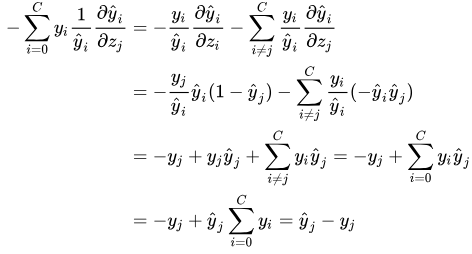但是对于梯度情况就不同了，我们可以像上面一样写出链式法则。

注意到这里的依赖于，依赖于和，等等。所以为了得到W的梯度，我们不能将看作常量。我们需要再次使用链式法则，得到的结果如下：

我们把每一时刻得到的梯度值累加，换句话说，在计算输出的每一步中都使用了。我们需要通过将时刻的梯度反向传播至时刻。

请注意，这与我们在深层前馈神经网络中使用的标准反向传播算法是完全相同的。关键的区别是，我们把每一时刻针对的不同梯度做了累加。在传统的神经网络中，我们不需要跨层共享权重，所以不需要求和。但在我看来，BPTT是应用于展开的RNN上的标准反向传播的另一个名字。就像反向传播一样，你也可以定义一个反向传递的delta向量，例如，

，其中

通过以上的推导，我们可以得出结论：

RNN很难训练的原因是：序列(句子)可能很长，可能是20个单词或更多，因此需要在许多层中进行反向传播。在实践中，许多人限定反向传播为有限的步骤（也是因为有梯度消失的问题存在）。

本部分参考文章：

【1】循环神经网络教程第三部分-BPTT和梯度消失 - 徐志强的文章 - 知乎 https://zhuanlan.zhihu.com/p/22338087

6. RNN的梯度消失和梯度爆炸

上面我们讲到的单向循环神经网络、双向循环神经网络和深度循环神经网络都是传统的循环神经网络。这些传统的循环神经网络在学习过程中容易出现梯度消失和梯度爆炸的现象。所谓的梯度消失是指：信息在反向传播时误差减小为0；而梯度爆炸是指：信息在反向传播时误差呈指数增长。下面，我们来深入的了解一下循环神经网络中产生梯度消失和梯度爆炸的本质原因。

6.1 梯度消失

RNN很难学到长范围的依赖-即相隔几步的词之间的联系。传统的RNN不能捕获长距离词之间的关系。要理解为什么，让我们先仔细看一下下面计算的梯度：

注意到也需要使用链式法则。例如，。注意到因为我们是用向量函数对向量求导数，结果是一个矩阵（称为Jacobian Matrix），矩阵元素是每个点的导数。我们可以把上面的梯度重写成：

可以证明上面的Jacobian矩阵的二范数（可以认为是一个绝对值）的上界是1。这很直观，因为激活函数把所有值映射到-1和1之间，导数值得界限也是1。

从图7中我们可以看到，tanh函数在x趋近于无穷大和无穷小时梯度值都为0。当这种情况出现时，我们就认为相应的神经元饱和。它们的梯度为0使得前面层的梯度也为0，因为前面层的梯度是由后面层的梯度连乘得到的。梯度中存在比较小的值，多个梯度相乘会使梯度值以指数级速度下降，最终在反向传播几步后就完全消失。比较远的时刻的梯度值为0，这些时刻的状态对学习过程没有帮助，导致你无法学习到长距离依赖。梯度消息问题不仅出现在RNN中，同样也出现在深度前向神经网中。只是RNN网络通常比较深，使得这个问题更加普遍。

6.2 梯度爆炸

RNN的学习依赖于我们的激活函数和网络初始参数，如果Jacobian矩阵中的值太大，会产生梯度爆炸而不是梯度消失问题。梯度消失比梯度爆炸受到了更多的关注有两方面的原因。其一，梯度爆炸容易发现，梯度值会变成NaN，导致程序崩溃。其二，用预定义的阈值裁剪梯度可以简单有效的解决梯度爆炸问题。梯度消失问题就不容易发现并且也不好处理，这也是梯度消失比梯度爆炸受到学术界更多关注的原因。

7. RNN的Long-Term依赖问题

上面我们讲到的循环神经网络梯度消失问题在RNN中会造成不能Long-Term依赖问题。

例如，考虑一个语言模型试图基于先前的词预测下一个词。如果我们要预测“the clouds are in the sky”，我们不需要其它更遥远的上下文信息就能预测出下一个词应该是sky。在这样的例子中，句子长度很小，相关信息和预测单词的距离很小，RNN可以学着去使用过去的信息。

但是，在大部分情况下我们需要更多的上下文信息。考虑预测这个句子中最后一个词：“I grew up in France… I speak fluent French.” 最近的信息表明下一个词可能是一种语言的名字，但如果我们想要找出是哪种语言，我们需要从更久远的地方获取France的上下文。相关信息和预测单词之间的距离完全可能是非常巨大的。而不幸的是，随着距离的增大，RNN会产生梯度消失的问题，造成不能够连接更长的上下文信息。

8. RNN的几种架构

由于RNN对于序列化的数据有很强的模型拟合能力，因此在不同的应用场景中可以对RNN组合形成不同的网络架构。

8.1 1 to 1

图8是普通的单个神经网络，由于图8模型过于简单，在实际的场景中没有什么应用价值。但是对于我们学习RNN的模型来说，它是最基本的也是最好理解的。

8.2 1 to N

这种情况有两种方式，一种是只在序列开始进行输入计算：

还有一种结构是把输入信息X作为每个阶段的输入：

这种1 to N的结构可以处理的问题有很多，比如图像标注，输入的X是图像的特征，而输出的y序列是一段句子。

8.3 N to 1

输入是一个序列，输出是一个单独的值而不是序列。这种结构通常用来处理序列分类问题。如输入一段文字判别它所属的类别，输入一个句子判断其情感倾向，输入一段文档并判断它的类别等等。具体如图11：

8.4 N to N

输入和输出序列是等长的。这种可以作为简单的Char RNN可以用来生成文章，诗歌，甚至是代码，非常有意思。

8.5 N to M

这种结构又叫Encoder-Decoder模型，也称之为Seq2Seq模型。在现实问题中，我们遇到的大部分序列都是不等长的。如机器翻译中，源语言和目标语言的句子往往并没有相同的长度。而Encoder-Decoder结构先将输入数据编码成一个上下文向量c，之后在通过这个上下文向量输出预测序列。

注意，很多时候只用上下文向量C效果并不是很好，而attention机制很大程度弥补了这点。seq2seq的应用的范围非常广泛，机器翻译，文本摘要，阅读理解，对话生成等等。

Reference

【1】 零基础入门深度学习(5) - 循环神经网络

地址：https://zybuluo.com/hanbingtao/note/541458

【2】 全面理解RNN及其不同架构

地址：https://zhuanlan.zhihu.com/p/34152808

【3】完全图解RNN、RNN变体、Seq2Seq、Attention机制

地址：https://zhuanlan.zhihu.com/p/28054589

【4】循环神经网络教程第一部分-RNN简介

地址：https://zhuanlan.zhihu.com/p/22266022

【5】Understanding LSTM Networks

地址：http://colah.github.io/posts/2015-08-Understanding-LSTMs/

【6】]YJango的循环神经网络——介绍 - YJango的文章 - 知乎

地址：https://zhuanlan.zhihu.com/p/24720659

【7】循环神经网络教程第三部分-BPTT和梯度消失 - 徐志强的文章 - 知乎

地址：https://zhuanlan.zhihu.com/p/22338087

【8】循环神经网络(RNN)介绍3：RNN的反向传播算法Backpropagation Through Time (BPTT) - 刘博的文章 - 知乎

地址：https://zhuanlan.zhihu.com/p/32930648

【9】神经网络梯度消失和梯度爆炸及解决办法展开全文RNN 深度学习 BPTT 梯度消失
• 本章中，将通过目前被称为循环神经网络（Recurrent Neural Networks，RNN）的一系列算法，为机器学习模型加入一个非常特别的维度（时间，即输入序列）。 7.1　按顺序解决问题——RNN 在前面的章节中，介绍了一系列...
了解了当前深度学习的发展状况，就已经接近了机器学习的前沿领域。本章中，将通过目前被称为循环神经网络（Recurrent Neural Networks，RNN）的一系列算法，为机器学习模型加入一个非常特别的维度（时间，即输入序列）。

7.1　按顺序解决问题——RNN

在前面的章节中，介绍了一系列的模型，从简单到复杂，这些模型都有一些共同的属性。

接受唯一且独立的输入。
输出数据维度唯一并固定。
输出仅依赖于当前输入的特性，与过去或之前的输入无关。
现实中，大脑处理信息片段的过程具有内在的结构和顺序，人类感知到的现象结构和顺序也会影响信息的处理过程。类似的例子包括语言理解（单词在句子中的顺序）、视频序列（视频中帧的顺序），以及语言翻译。这些都促成了新模型的诞生。最重要的一部分模型都利用到了RNN。

7.1.1　RNN的定义

RNN是一种输入和输出都有相应序列的人工神经网络（Artificial Neural Network，ANN）。正式的定义可以描述如下。

“循环神经网络表示固定维度的高维向量序列（称为隐藏状态），通过复杂的非线性函数与新的观察值结合。”

RNN具有很强的表达性，能够进行任意存储大小的计算，因此，通过配置，RNN在复杂的序列处理任务上可以达到非常优秀的性能。

序列类型

无论在输入还是输出范畴内，RNN都要基于序列模型工作。因此，可以采用所有可能的序列组合来解决不同种类的问题。如图7.1所示，描述了目前使用的主要序列结构，后续递归循环也会参考这些结构。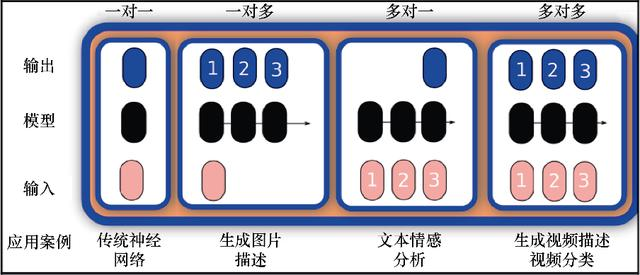图7.1　序列模型的种类

7.1.2　RNN的发展

RNN的起源与其他现代神经网络惊人地相似，可以追溯到20世纪80年代的Hopfield网络，但其在20世纪70年代就已有所发展。

循环网络的迭代结构如图7.2所示。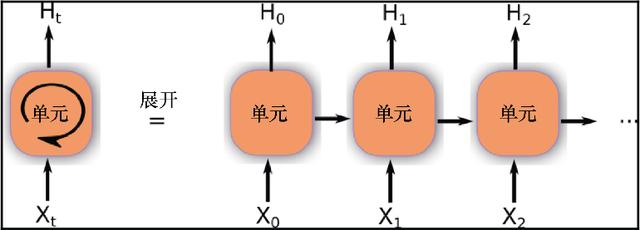图7.2　循环网络单元展开

经典RNN节点拥有连接到自身的一个循环链接，因此，可以将权值作为输入序列的一部分。另外，在图7.2的右侧，可以看到展开后的网络基于内部的模型产生一系列的输出。将当前输入事件以激活的形式保存（短期记忆，与长期记忆相反，体现在权重的缓慢变化上）。这对很多应用有重要的潜在意义，包括语音处理、音乐合成（例如Mozer，1992）、自然语言处理（Natural Language Processing，NLP）以及其他众多领域。

1．训练方法——后向传播

在研究了相当数量的模型之后，有可能会观察到这些模型训练步骤的一些共有模式。

对循环神经网络来说，常用的损失最小化技术是著名的后向传播算法的变种，后向传播算法（Backpropagation Through Time，BPTT）采用将所有输入时间步展开的方式工作。每个时间步包括输入时间步、整个网络的一个副本、一个输出。计算并累加每个时间步的损失，最后将整个网络卷起，并更新权值。

从空间上看，循环神经网络展开后的每个时间步可以看作是单独的一层，每个时间步之间存在依赖关系，每个时间步的输出都是其下一个时间步的输入。这导致了复杂的训练性能需求，因此，诞生了时间截断的反向传播算法。

下面的伪代码描述了整个过程。

Unfold the network to contain k instances of the cell
While (error < ε or iteration>max):
x = zeros(sequence_legth)
for t in range (0, n-sequence_length)  # initialize the weights
copy sequence_length input values into the input x
p = (forward-propagate the inputs over the whole unfolded
network)
e = y[t+k] - p;            # calculate error as target
- prediction
Back-propagate the error e, back across the whole unfolded
network
Sum the weight changes in the k model instances together.
Update all the weights in f and g.
x = f(x, a[t]);    # compute new input for the next
time- step

2．传统RNN的主要问题——梯度爆炸和消失

事实证明，训练RNN网络是很困难的，特别是对复杂的长距离问题——通过正确的配置，RNN或许是最有用的。由于潜在优势还未发挥出来，因此解决RNN训练难度的方法就显得尤为重要。

目前广泛用于学习短期记忆输入内容的算法需要耗费大量的时间，有时甚至无法工作，特别当输入和相应信号的最小时间间隔很长时。虽然理论上很迷人，但通过传统前向网络，现有的方法在实践中没有优势。

RNN的一个主要问题出现在后向传播阶段。考虑到其循环特性，损失后向传播的步数相当于一个深度非常深的网络。这种梯度的级联计算可能会导致在最后阶段梯度值非常小，或者相反，导致参数漫无边界的不断增大。这种现象被称为梯度消失和爆炸。这也是导致产生LSTM的原因之一。

传统BPTT的问题是损失信号在后向传播过程中要么爆炸式增加，要么消失——后向传播损失随着时间的变化指数性地依赖于权值大小。这有可能会导致权值振荡，或者耗费大量时间，甚至根本无法工作。

为解决梯度消失或爆炸问题，研究者进行了大量不同的尝试，最终在1997年，Schmidhuber和Sepp发布了一篇关于RNN和LSTM的基础研究论文，为这一领域的发展铺平了道路。

7.2　LSTM

LSTM是RNN的基本步骤，它将长期依赖性地引入RNN单元。展开后的单元包括两种不同的参数：一种是长期状态，另一种表示短期记忆。

每步之间，长期状态会遗忘不太重要的信息，同时增加经过过滤的来自短期记忆的事件信息，并将两者结合后输出到后续步骤。

LSTM在其可能的应用中是非常灵活的，与GRU一样，都是被广泛应用的循环模型，GRU将在后面介绍。为方便理解LSTM的工作过程，下面会将其按组成单元进行分解。

7.2.1　门和乘法运算

LSTM有两类基本的功能：记住当前重要的事情，缓慢遗忘过去不重要的事情。有哪种机制可以实现这种过滤功能？这种运算被称为门运算。

门运算的基本元素包括一个多元向量和一个过滤向量，过滤向量与输入值点乘，以允许或拒绝某些输入元素被传输。如何调整门过滤器呢？这个多元控制向量（在图7.3中用箭头表示）通过sigmoid激活函数与神经网络层相连。控制向量通过sigmoid激活函数后将产生类似二进制的输出。

在图7.3中，用一系列开关表示门。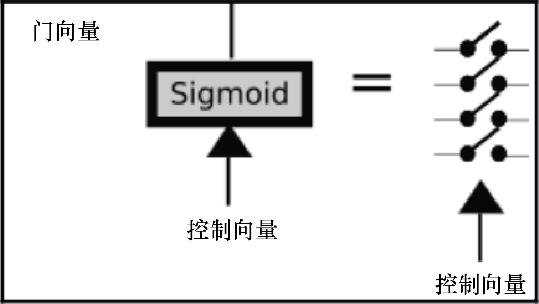图7.3　LSTM门

此过程中另外一个重要的部分是乘法运算，将训练过的过滤器正规化，将输入与门向量相乘。图7.4中的箭头图标表示过滤后的信号传输方向。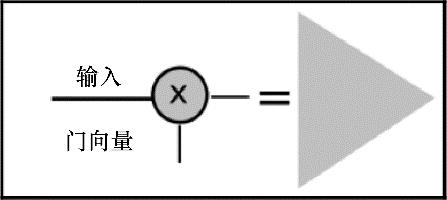图7.4　门相乘运算

下面进一步具体讲述LSTM各单元的细节。

LSTM包含3个门，用于保护和控制单元状态：一个位于数据流的起始位置，另一个位于中间，最后一个位于单元信息边界的尾部。这个运算过程允许丢弃低重要性（期望不重要）的状态数据，并将新的数据（期望重要）与当前状态结合。

图7.5显示了LSTM单元内部运算的概念。以下信息将被作为输入。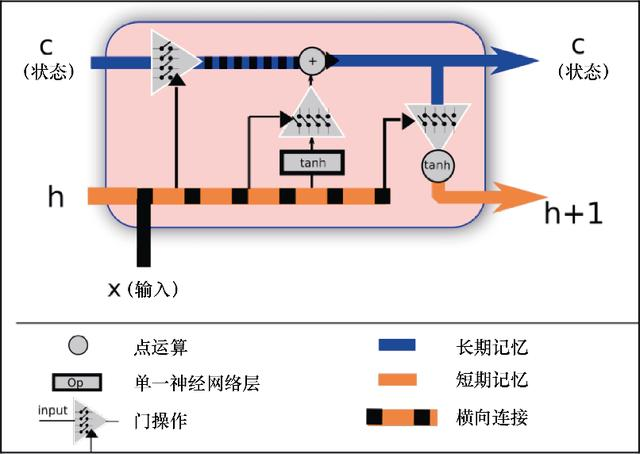图7.5　LSTM单元及其组件

单元状态，保存有长期信息，因为其装载了自初始单元以来的训练优化后的权值。
短期状态，h(t)，在每次迭代时直接与当前状态结合，所以对最新的输入值有重要影响。
下面，介绍LSTM单元的数据流，以便更好地理解LSTM单元中不同的门和运算是如何协同工作的。

7.2.2　设置遗忘参数（输入门）

在这一阶段，将来自短期记忆的值和输入结合（见图7.6），结果输出给一个用多变量Sigmoid函数表示的二进制函数。根据输入和短期记忆值，Sigmoid函数会过滤掉用单元状态权值表示的长期知识。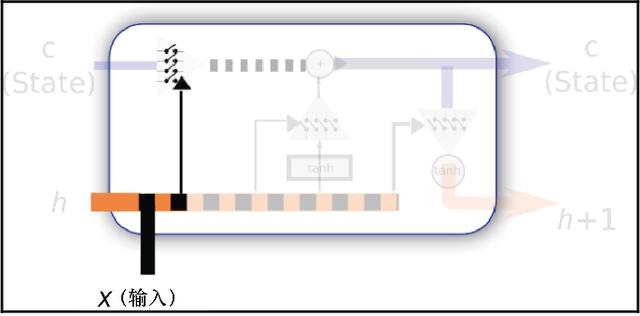图7.6　状态遗忘参数设置

7.2.3　设置保持参数

下面通过设置过滤器，允许或拒绝新数据与短期记忆结合后的值进入单元的半永久状态，如图7.7所示。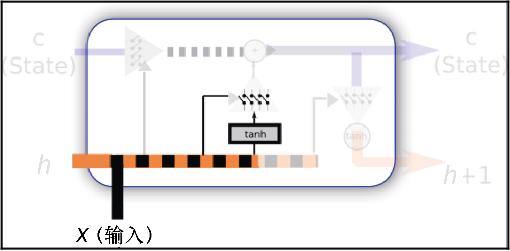图7.7　短期值选择性设置

在这一阶段，将决定多少全新和半新的信息结合并进入单元新的状态。

7.2.4　修改单元

在序列的这一部分，通过配置过的信息过滤器传输相关信息，最终将得到更新后的长期状态值。

为了将新的信息和短期信息归一化，将新的输入和短期状态通过tanh激活函数输入神经网络。这将确保输入的新信息归一化到[−1，1]范围，如图7.8所示。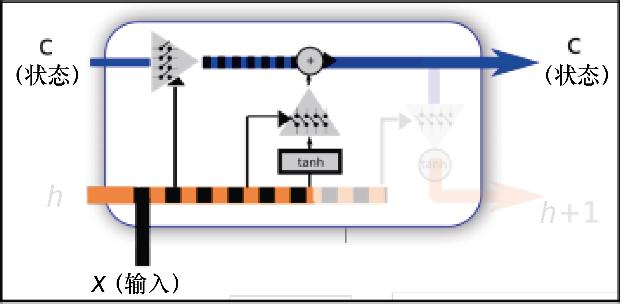图7.8　状态持续改变的过程

7.2.5　输出过滤后的单元状态

接下来介绍短期状态。这里也需要用到经过新数据和之前的短期状态配置的过滤器，长期状态可以通过过滤器与tanh函数点乘，再次将信息归一化到[−1，1]的范围内，如图7.9所示。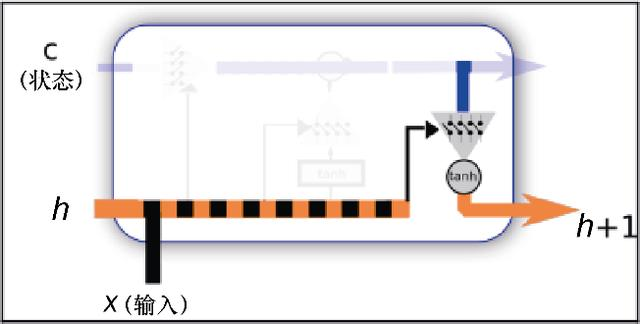图7.9　新的短期状态产生过程

7.3　采用电能消耗数据预测单变量时间序列

在接下来的例子中，将解决回归领域的一个问题。因此，使用两个LSTM创建一个多层RNN。即将进行的回归类型属于多对一型，因为网络将收到电能消耗的数据序列，并尝试基于前面4个记录数据预测接下来的值。

本案例中采用的数据集来自一个家庭某段时间的电能消耗记录。可以推断，家庭电能消耗有很多规律可以遵循（比如居住者采用微波炉准备早餐以及整天使用计算机时，电能消耗将增加。下午会有一点下降，然后晚上会增加，因为所有灯都会打开，最后在零点左右开始下降，因为这时居住者已经休息了）。

首先加载必要的库文件，并初始化相应的环境变量，代码如下。

%matplotlib inline
%config InlineBackend.figure_formats = {'png', 'retina'}

import numpy as np
import pandas as pd
import tensorflow as tf
from matplotlib import pyplot as plt

from keras.models import Sequential
from keras.layers.core import Dense, Activation
from keras.layers.recurrent import LSTM
from keras.layers import Dropout

Using TensorFlow backend.

数据集的描述和加载

“数据集没有缺失值。

“数据是每15分钟的kW值。除以4可以将数据转换为kWh数据。每列代表一个客户。有些客户是2011年之后创建的。这种情况下，将之前的电能消耗数据默认为是0。

“所有时间标签为葡萄牙的小时数。每天有96条记录（24×15）。每年3月份时间切换日（这一天只有23小时），上午1:00～2:00之间的所有记录为0。每年十月也是时间切换日（这一天有25小时），上午1:00～2:00之间的电能消耗按2个小时统计。”

为简化模型描述，采用一个客户完整的测量数据，并将其转换成标准的CSV格式。数据位于本章代码目录下的data子目录。

因此，首先从数据集中加载同一个家庭的1 500条电能消耗记录。

df = pd.read_csv("data/elec_load.csv", error_bad_lines=False)
plt.subplot()
plt.legend(handles=[plot_test])

图7.10显示了即将应用于模型的数据子集。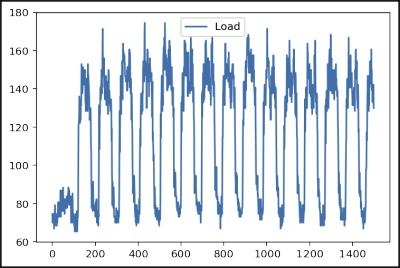图7.10　前1 500条样本数据

查看这些数据的图示（采用了最开始的1 500条样本），可以看到初始的状态，这可能是刚开始测量时的记录值，然后可以看到清晰的电能消耗的高低周期变化。通过简单的观察，可以看到每个周期大致有100条记录，非常接近数据集所拥有的每天96条记录。

数据集预处理

为了确保后向传播算法能更好地覆盖数据集，需要将输入数据归一化。采用经典的缩放和对中技术，减去平均值，并使用最大值的floor()函数对数据缩放。使用Pandas的describe()方法得到需要的值。

print(df.describe())
array=(df.values - 145.33) /338.21
plt.subplot()
plt.legend(handles=[plot_test])

count  140256.000000
mean      145.332503
std        48.477976
min         0.000000
25%       106.850998
50%       151.428571
75%       177.557604
max       338.218126

图7.11所示为归一化后的数据。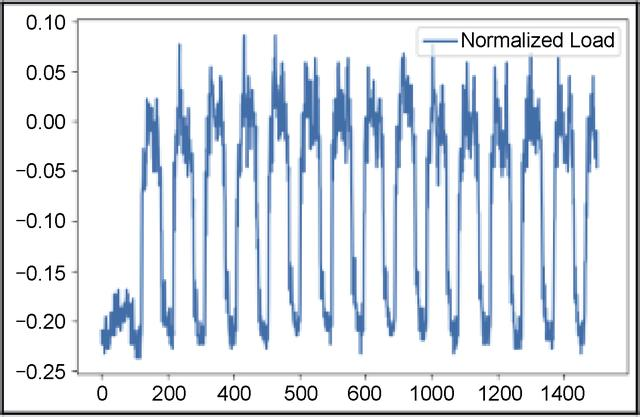图7.11　归一化后的数据

在这一步中，将准备输入数据集，需要输入值x（前面5个值）以及对应的输入值y（5个时间步后的值）。然后，将其中13 000个数据分配给训练数据集，将随后的1 000个样本分配给测试集。

listX = []
listy = []
X={}
y={}

for i in range(0,len(array)-6):
listX.append(array[i:i+5].reshape([5,1]))
listy.append(array[i+6])

arrayX=np.array(listX)
arrayy=np.array(listy)

X['train']=arrayX[0:13000]
X['test']=arrayX[13000:14000]

y['train']=arrayy[0:13000]
y['test']=arrayy[13000:14000]

下面开始创建模型，模型拥有两个LSTM和一个位于最后的dropout层。

#Build the model
model = Sequential()

return_sequences=False))

model.compile(loss="mse", optimizer="rmsprop")

运行模型并调整权值。模型将使用数据集中8%的数据作为验证集。

#Fit the model to the data

model.fit(X['train'], y['train'], batch_size=512, epochs=10,
validation_split=0.08)

Train on 11960 samples, validate on 1040 samples
Epoch 1/10
11960/11960 [==============================] - 41s - loss: 0.0035 -
val_loss: 0.0022
Epoch 2/10
11960/11960 [==============================] - 61s - loss: 0.0020 -
val_loss: 0.0020
Epoch 3/10
11960/11960 [==============================] - 45s - loss: 0.0019 -
val_loss: 0.0018
Epoch 4/10
11960/11960 [==============================] - 29s - loss: 0.0017 -
val_loss: 0.0020
Epoch 5/10
11960/11960 [==============================] - 30s - loss: 0.0016 -
val_loss: 0.0015
Epoch 6/10
11960/11960 [==============================] - 28s - loss: 0.0015 -
val_loss: 0.0013
Epoch 7/10
11960/11960 [==============================] - 43s - loss: 0.0014 -
val_loss: 0.0012
Epoch 8/10
11960/11960 [==============================] - 37s - loss: 0.0013 -
val_loss: 0.0013
Epoch 9/10
11960/11960 [==============================] - 31s - loss: 0.0013 -
val_loss: 0.0012
Epoch 10/10
11960/11960 [==============================] - 25s - loss: 0.0012 -
val_loss: 0.0011

<keras.callbacks.History at 0x7fa435512588>

重新调整后，查看模型预测的值，与真实值比较，真实值并没有参与模型训练，进一步理解模型是如何预测并生成样本家庭的行为的。

# Rescale the test dataset and predicted data

test_results = model.predict( X['test'])

test_results = test_results * 338.21 + 145.33
y['test'] = y['test'] * 338.21 + 145.33

plt.figure(figsize=(10,15))
plot_predicted, = plt.plot(test_results, label='predicted')

plot_test, = plt.plot(y['test'] , label='test');
plt.legend(handles=[plot_predicted, plot_test]);

图7.12所示为最终经回归后的数据。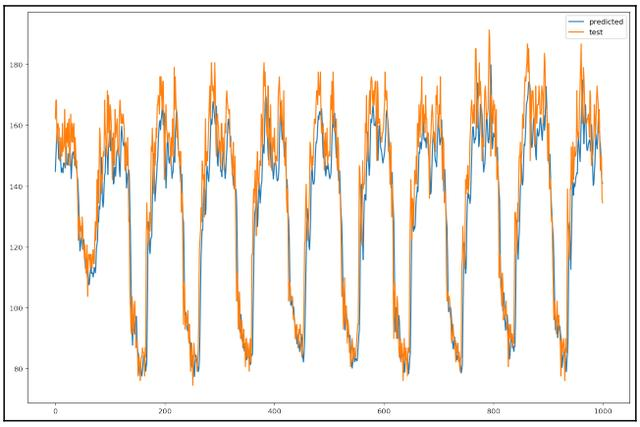图7.12　最终经回归后的数据

7.4　小结

在本章中，视野进一步得到扩展，将时间这一重要维度添加到泛化的元素中。基于真实数据，学习了如何应用RNN解决实际的问题。

如果读者已经掌握了所有可能的选项，也可以进一步了解很多种类的模型。

在随后的章节中，将讨论其他重要的架构，这些架构经过训练，可用于生成更聪明的元素，例如，将著名画家的风格转移到一幅图片上，甚至玩视频游戏！请继续阅读后续关于强化学习和生成对抗网络的章节。

本文摘自《机器学习开发者指南》。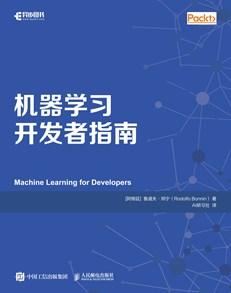本书将带领读者学习如何实施各种机器学习技术及其日常应用的开发。本书分为9章，从易于掌握的语言基础数据和数学模型开始，向读者介绍机器学习领域中使用的各种库和框架，然后通过有趣的示例实现回归、聚类、分类、神经网络等，从而解决如图像分析、自然语言处理和时间序列数据的异常检测等实际问题。

展开全文rnn
• 应用循环神经网络的过程中，还是会有些地方疑惑，所以还是要回归下问题的本质。学而不思则惘，思而不学则怠。。 1. 循环神经网路简介 首先循环神经网络的主要用途是处理和预测序列数据。在之前的全链接神经网络...LSTM dropout
•   在传统的全连接神经网络中，从输入层到隐藏层再到输出层，每一层之间的节点是无连接的，因为输入和输出是独立的，所以这种普通的神经网络对于序列数据的处理是无能为力的。而现实中，绝大多数的数据都是序列数据...RNN 情感分析
• 深度学习笔记(四)：循环神经网络的概念，结构和代码注释本文的概念和结构部分摘自循环神经网络惊人的有效性（上），代码部分来自minimal character-level RNN language model in Python/numpy 我对代码做了详细的注神经网络 深度学习 RNN
• DL之RNN：循环神经网络RNN的简介、应用、经典案例之详细攻略 目录 循环神经网络RNN的简介 1、RNN的分类 1、RNN的常见算法分类 2、RNN的三种分类 2、循环神经网络RNN的重要进展 2.1、1982年反馈神经网络...
• 作者：徐志强 ... 来源：知乎 著作权归作者所有。商业转载请联系作者获得授权，非商业转载请注明出处。 ...本篇教程的代码在Github上。...循环神经网络教程第二部分-用python，numpy，theano实现一个RNN
• 循环神经网络以及 LSTM 一、循环神经网络 1.1 RNN 简介概述 在前面讲到的 DNN 以及 CNN 等算法都是前向反馈的网络，即给一个输入，得到一个输出，而不考虑输入的序列间的关系，而本节我们要讨论的 RNN...LSTM
• 1.1、循环神经网络 循环神经网络（Recurrent Neural Network, RNN）是一类以序列数据为输入，在序列的演进方向进行递归且所有节点（循环单元）按链式连接的递归神经网络。 卷积网络的输入只有输入数据X，而循环...文本分类
• 文章目录通俗LSTM长短时记忆循环神经网络介绍LSTM图解处理流程流程图解总结说明PyTorch实战参考资料 版权声明：本文为博主原创文章，转载请注明原文出处！ 写作时间：2019-03-02 18:20:11 本文部分图片素材来自...LSTM 深度学习 PyTorch
• • 循环神经网络（rnn）• RNN简介• Rnn基本结构• 双向rnn基本结构• rnn梯度消失问题• RNN应用举例 • RNN简介• 循环神经网络（RNN）能够从序列和时序数据中学习特征和长期依赖关系。• 循环神经网络...RNN
• RNN（循环神经网络）1 RNN(循环神经网络)的主要应用 RNN主要应用在处理和预测序列数据。主要应用在语音识别，语言模型，机器翻译等有关时间序列分析的方面。 对于RNN最重要的就是时刻，它会根据每个时刻的 输入结合...RNN
• gitbook阅读地址：循环神经网络--实现LSTM · 超智能体 梯度消失和梯度爆炸 网络回忆：在《循环神经网络——介绍》中提到循环神经网络用相同的方式处理每个时刻的数据。 动态图： 数学公式： ...
•视觉注意力
• ## 简述循环神经网络

千次阅读 2018-08-15 14:01:32
下面是 RNN 应用最多的领域。 1. 语言建模和文本生成 给出一个词语序列，试着预测下一个词语的可能性。这在翻译任务中是很有用的，因为最有可能的句子将是可能性最高的单词组成的句子。 2. 机器翻译 将文本内容...RNN
• 本来想继续学习tensorflow图像方面的应用，但是循环神经网络的某一个应用吸引到了我，所以就先学学这个循环神经网络。 2、用处 前面学习的全连接神经网络或者卷积神经网络，网络结构都是从输入层，到隐含...tensorflow RNN
• 文章目录使用循环神经网络做手写数字识别思路分析PyTorch实现 版权声明：本文为博主原创文章，转载请注明原文出处！ 写作时间：2019-03-02 21:36:12 使用循环神经网络做手写数字识别 思路分析 做图像识别的使用卷积...PyTorch LSTM MNIST 手写识别
• 作者 | 李理 目前就职于环信，即时通讯云平台和全媒体智能客服平台，在环信从事智能客服和智能机器人相关工作，致力于用深度学习来提高...下面的系列文章会首先会介绍 vanilla RNN 的代码，希望读者能够通过代码...
• 作者：jliang ... junliang 20190303 说明：以下所有代码使用版本TensorFlow1.4.0或1.12.0版本 import tensorflow as tf print(tf.__version__)...8. 循环神经网络 ...TensorFlow中实现LSTM结构的循环神经网络的前向...
• 当一个机器学习模型工作在诸如循环神经网络、LSTM-RNN、GRU等序列模型上时，它们可以生成输入文本的下一个序列。 PyTorch提供了一组功能强大的工具和库，这些工具和库为这些基于NLP的任务增添了动力。它不仅需要较少...
• 之前看的论文都是基于CNN在NLP上的应用，但其实深度学习与NLP结合的领域中应用最广的应该是RNN，因为文本可以直观地被表示为输入序列，方便的被RNN处理，捕获其Long-Term依赖等信息，而且实际应用中也取得了很好的...自然语言处理 深度学习
• 专栏——TensorFlow学习笔记 ...循环神经网络（Recurrent Neural Network, RNN） 是一种适宜于处理 序列数据 的神经网络，被广泛用于语言模型、文本生成、机器翻译等。基础知识可以看一下这个英文...深度学习 RNN TensorFlow
• 本文重点讲述的是一种被称为attention的方法，有的人将其译为“聚焦”，但觉得这种翻译将原文对神经网络拟人化的手法给扔掉了，因此保留了原来的称谓。Attention，顾名思义，就是让神经网络对部分内容引起注意，而...深度学习
• 我们在深度学习与计算机视觉专栏中看过计算机通过卷积神经网络学会了识别图片的内容——模仿人类的看，而工业界大量的应用也证明了神经网络能让计算机学会听（比如百度的语音识别），于是大量的精力开始投向NLP领域...神经网络 自然语言处理 机器学习
• 从本专栏开始，作者正式研究Python深度学习、神经网络及人工智能相关...这篇文章将详细讲解循环神经网络RNN的原理知识，并采用TensorFlow实现手写数字识别的RNN分类案例及可视化呈现。基础性文章，希望对您有所帮助！人工智能 Python Keras RNN...